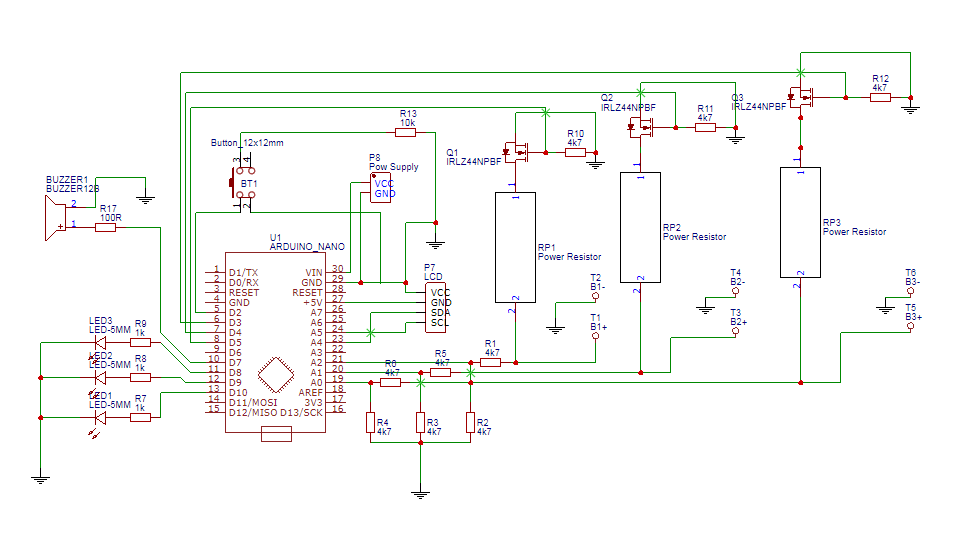# Help Modifying Diy 3x 1s battery capacity tester for higher voltage / current

Ive built a large amount of these diy 3x 18650 capacity testers I found on Instructables. They work great. Pcb Design and code had no problems.

Ive been looking for a simple diy load tester to use with 2s-7s battery packs, when it dawned on me that maybe I could Simply modify One of these capacity testers....

Im not good with programming but the arduino code seems like it should work without much modification... Besides setting the appropriate cut off voltage the only other problem that I see is the voltage dividers on A0, A1, A2 would have to be changed.

basically my first question is what resistor values would I need to use for the voltage dividers in order to use higher voltage batteries and if anything in the code also have to be changed? ideally I would like to be able to test 2's batteries in one slot, 4s in another and 7s in the third. not all at once but have it set up so if I want to test a 2s or 4S or 7s I would attach it to the appropriate spot.

so if someone could please advise me only appropriate resistor values for the voltage dividers and if anything in the code needs to be modified for this to work I would greatly appreciate it.

Thank You

below I have attached the schematic and code for the Arduinowell I guess I can't post the code for the Arduino because it's longer than 9000 characters. So here is a link to paste bin. https://pastebin.pl/view/b910326f

The power resistors determine the maximum rate you can draw power from the battery. The number of Amps drawn is the Voltage of the battery divided by the resistance in Ohms. Multiply the Voltage by the Amps to get Watts to see what the minimum power rating of the resistors must be.

The existing voltage dividers use 4.7k Ohms on both sides. That divides the voltage in half. Since the cell voltage shouldn't be more than 4.2V this brings the reading down to 2.1V or lower. Unless the Arduino Nano is an 8MHz model with a 3.3V regulator there was no need for a voltage divider.

For more cells in series, multiply 4.2V by the number of cells to get MaxV. Divide MaxV by 5V (Vcc) to determine the minimum voltage divider. For a 7S pack, MaxV is 29.4. 29.4 / 5 = 5.88 so figure a divisor of 6 or more. A total resistance around 10k Ohm is good so divide that by 6 to get the ground-side resistor: 1.666...k. Subtract that from 10k to get the battery-side resistor: 8.333...k. Those aren't standard resistor values. The nearest E6 standard values are 1.5k and 10.0k for a divisor of 7.666... (11.5/1.5). Using the more precise E12 series you can get 1.5k and 8.2k for a divisor of 6.4666... (9.7/1.5) Resistors have a specified tolerance (typically 10%, 5%, or 1%) so measure your resistors and use the measured values for calculations.